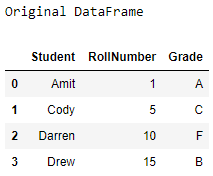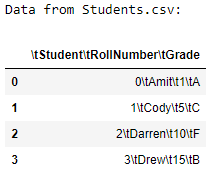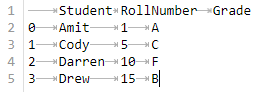# Pandas – DataFrame to CSV file using tab separator

• Last Updated : 27 Aug, 2021

Let’s see how to convert a DataFrame to a CSV file using the tab separator. We will be using the to_csv() method to save a DataFrame as a csv file. To save the DataFrame with tab separators, we have to pass “\t” as the sep parameter in the to_csv() method.

Approach :

Attention geek! Strengthen your foundations with the Python Programming Foundation Course and learn the basics.

To begin with, your interview preparations Enhance your Data Structures concepts with the Python DS Course. And to begin with your Machine Learning Journey, join the Machine Learning - Basic Level Course

1. Import the Pandas and Numpy modules.
2. Create a DataFrame using the DataFrame() method.
3. Save the DataFrame as a csv file using the to_csv() method with the parameter sep as “\t”.
4. Load the newly created CSV file using the read_csv() method as a DataFrame.
5. Display the new DataFrame.

## Python3

 `# importing the modules``import` `pandas as pd``import` `numpy as np` `# creating a DataFrame``students ``=` `{``'Student'``: [``'Amit'``, ``'Cody'``,``                        ``'Darren'``, ``'Drew'``],``            ``'RollNumber'``: [``1``, ``5``, ``10``, ``15``],``            ``'Grade'``: [``'A'``, ``'C'``, ``'F'``, ``'B'``]}``df ``=` `pd.DataFrame(students,``                  ``columns ``=``[``'Student'``, ``'RollNumber'``,``                            ``'Grade'``])``# displaying the original DataFrame``print``(``"Original DataFrame"``)``print``(df)` `# saving as a CSV file``df.to_csv(``'Students.csv'``, sep ``=``'\t'``)` `# loading the CSV file``new_df ``=` `pd.read_csv(``'Students.csv'``)` `# displaying the new DataFrame``print``(``'Data from Students.csv:'``)``print``(new_df)`

Output :The contents of the Students.csv file are :My Personal Notes arrow_drop_up